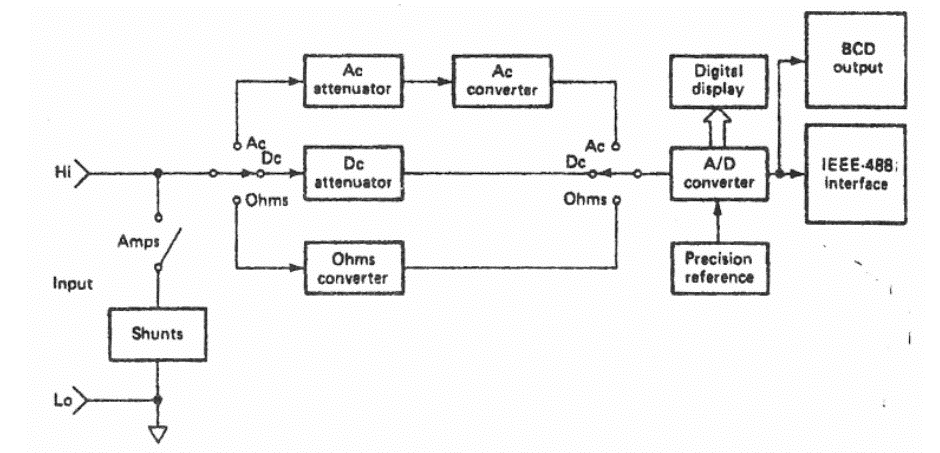Question:
Published on: 23 June, 2022

Write short note on

• Digital Multimeter
• Piezoelectric Transducer

Digital Multimeter: While most analog meters require no power supply, give a better visual indication of trends and changes, suffer less from electric noise and isolation problems, and, are simple and inexpensive, digital meters offer higher accuracy and input impedance, unambiguous readings at greater viewing distances, smaller size, and a digital electrical output (for interfacing with external equipment) in addition to visual readout. The main part of most of the digital multimeter (DMMs) is the analog to digital converter (A/D) which converts an analog input signal to a digital output. While specifications may vary, virtually such multimeters are developed around the same block diagram.Since the DMM is a voltage sensing meter; current is converted to volts by passing it through a precision low resistance shunt while ac is converted to dc at the AC converter by employing rectifiers and filters. Most of the AC converters detect the peak value of the signal and are calibrated to give the rms value of a sine wave. However, some measures the mean of the rectified signal such as the digital multimeter Agilent 34401A. Finally, this dc level is applied to the A/D converter to obtain the digital information.

For resistance measurement, the meter includes a precision low current source that is applied across the unknown resistor. Then the dc voltage drop across the resistor, which is proportional to the value of the unknown resistor, is measured.

For AC measurements, the digital multimeter is a true rms instrument that it measures true rms value of any periodic signal.

Piezoelectric effect:- Piezoelectricity is the charge that accumulates in certain solid materials such as crystals, certain ceramics etc. in response to applied mechanical stress. Due to displacement of charges, an electric potential appears across certain surfaces of the crystal. The word piezoelectricity means electricity resulting from pressure. Piezo is a Greek word which means to squeeze or press.

The Piezoelectric effect is a reversible process i.e. conversely, if a varying potential is applied to the proper a (is of the crystal, it will change the dimensions of the crystal, thereby deforming it. This effect is known as piezoelectric effect. Elements exhibiting piezoelectric effect are called as electro resistive elements. A piezoelectric element used for converting mechanical motion into electrical signal can be considered as a charge generator and capacitor. Mechanical deformation generates a charge and this charge appears as a voltage across the electrodes.

The voltage is $$E= \frac{Q}{C}$$

Piezoelectric effect is direction sensitive i.e. a tensile force produces a voltage of one polarity while compressible voltage produces a voltage of opposite polarity. Piezoelectric effect can be made to respond to mechanical deformations of the materials in many different modes- thickness expansion, transverse expansion, thickness shear, face shear. The mode of motion affected depends on shape of body relative to crystal axis and location of electrodes.